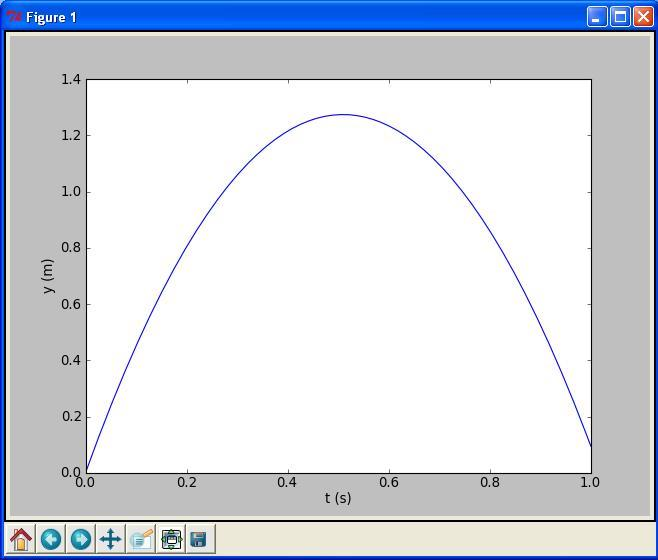$$\newcommand{\Oof}{\mathcal{O}(#1)} \newcommand{\F}{\boldsymbol{F}} \newcommand{\J}{\boldsymbol{J}} \newcommand{\x}{\boldsymbol{x}} \renewcommand{\c}{\boldsymbol{c}}$$

# A Python program with vectorization and plotting

We return to the problem where a ball is thrown up in the air and we have a formula for the vertical position $$y$$ of the ball. Say we are interested in $$y$$ at every milli-second for the first second of the flight. This requires repeating the calculation of $$y=v_0t-0.5gt^2$$ one thousand times.

We will also draw a graph of $$y$$ versus $$t$$ for $$t\in [0,1]$$. Drawing such graphs on a computer essentially means drawing straight lines between points on the curve, so we need many points to make the visual impression of a smooth curve. With one thousand points, as we aim to compute here, the curve looks indeed very smooth.

In Python, the calculations and the visualization of the curve may be done with the program ball_plot.py, reading

from numpy import linspace
import matplotlib.pyplot as plt

v0 = 5
g = 9.81
t = linspace(0, 1, 1001)

y = v0*t - 0.5*g*t**2

plt.plot(t, y)
plt.xlabel('t (s)')
plt.ylabel('y (m)')
plt.show()


This program produces a plot of the vertical position with time, as seen in Figure 1. As you notice, the code lines from the ball.py program in the chapter A Python program with variables have not changed much, but the height is now computed and plotted for a thousand points in time!

Let us take a look at the differences between the new program and our previous program. From the top, the first difference we notice are the lines

from numpy import *
from matplotlib.pyplot import *


You see the word import here, so you understand that numpy must be a library, or module in Python terminology. This library contains a lot of very useful functionality for mathematical computing, while the matplotlib.pyplot module contains functionality for plotting curves. The above import statement constitutes a quick way of populating your program with all necessary functionality for mathematical computing and plotting. However, we actually make use of only a few functions in the present program: linspace, plot, xlabel, and ylabel. Many computer scientists will therefore argue that we should explicitly import what we need and not everything (the star *):

from numpy import linspace
from matplotlib.pyplot import plot, xlabel, ylabel


Others will claim that we should do a slightly different import and prefix library functions by the library name:

import numpy as np
import matplotlib.pyplot as plt
...
t = np.linspace(0, 1, 1001)
...
plt.plot(t, y)
plt.xlabel('t (s)')
plt.ylabel('y (m)')


We will use all three techniques, and since all of them are in so widespread use, you should be familiar with them too. However, for the most part in this book we shall do

from numpy import *
from matplotlib.pyplot import *


for convenience and for making Python programs that look very similar to their Matlab counterparts.

The function linspace takes $$3$$ parameters, and is generally called as

linspace(start, stop, n)


This is our first example of a Python function that takes multiple arguments. The linspace function generates n equally spaced coordinates, starting with start and ending with stop. The expression linspace(0, 1, 1001) creates 1001 coordinates between 0 and 1 (including both 0 and 1). The mathematically inclined reader will notice that 1001 coordinates correspond to 1000 equal-sized intervals in $$[0,1]$$ and that the coordinates are then given by $$t_i = i/1000$$ ($$i = 0, 1, ..., 1000$$).

Figure 1: Plot generated by the script ball_plot.py showing the vertical position of the ball at a thousand points in time.The value returned from linspace (being stored in t) is an array, i.e., a collection of numbers. When we start computing with this collection of numbers in the arithmetic expression v0*t - 0.5*g*t**2, the expression is calculated for every number in t (i.e., every $$t_i$$ for $$i = 0, 1,..., 1000$$), yielding a similar collection of 1001 numbers in the result y. That is, y is also an array.

This technique of computing all numbers "in one chunk" is referred to as vectorization. When it can be used, it is very handy, since both the amount of code and computation time is reduced compared to writing a corresponding for or while loop (the chapter Basic constructions) for doing the same thing.

The plotting commands are simple:

1. plot(t, y) means plotting all the y coordinates versus all the t coordinates
2. xlabel('t (s)') places the text t (s) on the $$x$$ axis
3. ylabel('y (m)') places the text y (m) on the $$y$$ axis
At this stage, you are strongly encouraged to do Exercise 4: Volumes of three cubes. It builds on the example above, but is much simpler both with respect to the mathematics and the amount of numbers involved.